StatLect

# Unadjusted sample variance

The unadjusted sample variance measures the average dispersion of a sample of observations around their mean.

It is computed by averaging the squared deviations from the mean.## Synonyms

It is also often called biased sample variance, because, under standard assumptions, it is a biased estimator of the population variance.

## Definition

It is defined as follows:

Definition Givenobservations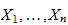, their unadjusted sample variance iswhereis their sample mean:## Example

Suppose that the sample consists of the following observations: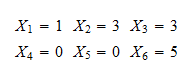We arrange the observations into a table and use the table to do all the necessary calculations: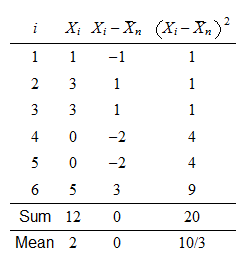Thus, the sample variance is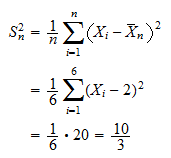## The bias

Suppose thatare independent realizations of random variables having the same meanand the same variance.

It can be proved (see Variance estimation) that the unadjusted sample varianceis a biased estimator of, that is,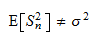where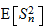is the expected value of.

## The cause of the bias

The cause of the bias is that we use the sample meaninstead of the true meanin the calculation.

Ifwere known and used in the formula in place of, then the resulting estimator would be unbiased.

## How to correct the bias

In order to obtain an unbiased estimator of the true variance, we need to perform a so-called degrees of freedom adjustment: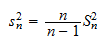The estimatoris unbiased (see Adjusted sample variance).

## Maximum likelihood estimator

If the observationsare not only independently and identically distributed, but they are also normal, then the biased sample variance coincides with the maximum likelihood estimator of the variance(see Maximum likelihood estimation of the parameters of a normal distribution).

## More details

The lecture entitled Variance estimation provides a thorough introduction to the concept of unadjusted sample variance, including a detailed analysis of its statistical properties (e.g., its bias as an estimator of the population variance).

## Keep reading the glossary

Previous entry: Type II error

Next entry: Unbiased estimator